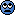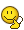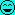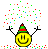Water inside a pot of copper (Thermodynamics problem)

ValeForce46

Homework Statement
A pot of Cu of mass $2.0 Kg$ (including the lid) is at a temperature of $150 °C$. You pour inside the pot $0.10 Kg$ of water at a temperature of $25 °C$ and you close immediately the lid, so that the gas can't go out of the pot. Find the final temperature of the pot and its contents, and determine in which phase (or mixture of phase) the water is. Assume there's no heat loss to the environment.
[specific heat Cu $c_{cu}=0.39 \frac{J}{g °C}$ specific heat water $c_{H2O}=4.19 \frac{J}{g °C}$ latent heat of vaporization water $L_{H2O}=2256 \frac{J}{g}$]
Homework Equations
Heat: $Q=mc(T_f-T_0)$
$Q=mL_{H2O}$
I tried to write down the equation of heat exchanged:
$Q_{Cu}=m_{cu}*c_{cu}*(T_f-T_0)$ for the pot and $T_f$ is my unknown.
$Q_{H2O}=m_{H2O}*c_{H2O}*(T_e-T_{H2O})$ where $T_e=100 °C$ is the temperature of vaporization of the water.
But $Q_{H2O}$ is not all the exchanged heat by the water, because a part (or all) of water became vapour.
How do I manage this situation?

•Delta2
Related Introductory Physics Homework Help News on Phys.org

collinsmark

Homework Helper
Gold Member
In problems such as this one, you need to play with the numbers to figure out which scenario the final state of the contents are in. There are three possibilities:

Scenario 1: The final temperature of the contents are less than $100^\circ \ \mathrm{C}$. Here, $T_f$ is your unknown, but you don't have to worry about water becoming vapor because all of it (practically) is still liquid.

Scenario 2: Some of the water, but not all of it, boiled away into the gas phase. Here, the amount of water that transitioned from liquid to gas is your unknown. However, $T_f$ is not an unknown. $T_f$ is $100 ^\circ \ \mathrm{C}$.

Scenario 3: All of the water transitioned to the gas phase. Here, $T_f$ is your unknown, and it's greater than or equal to $100^\circ \ \mathrm{C}$. But unlike scenario 1, here you need to account for the energy of all of the water transitioning from liquid to gas phase. But that's not an unknown, since you know exactly how much water transitioned from liquid to gas (all of it).

When solving problems like this, you need to start with an assumption, and see if it makes sense.

For example, start by assuming that you are in scenario 1. Solve for the final temperature. If the final temperature is above $100^\circ \ \mathrm{C}$, then your initial assumption doesn't make sense and you can cross scenario 1 off the list and move on to one of the remaining two remaining scenarios, and so on.

••Delta2 and ValeForce46

ValeForce46

Thank you a lot for your reply, you were amazingly clear. I'll show my results, but first I have a doubt I forgot to ask before, hope this is not too dumb: if the water evaporates, does its specific heat change or the water vapour has the same specific heat? Why?

Scenario 1: All the water is still a liquid. (So $T_f$ MUST be less than $100 °C$)
$Q_{Cu}=m_{Cu}*c_{Cu}*(T_f-T_{Cu})$
$Q_{H2O}=m_{H2O}*c_{H2O}*(T_f-T_{H2O})$
From $Q_{Cu}+Q_{H2O}=0$ I get $$T_f=106 °C$$
and this doesn't makes sense.

Scenario 2: A part of the water evaporates. ($T_f$ MUST be $100 °C$)
$Q_{Cu}=m_{Cu}*c_{Cu}*(T_f-T_{Cu})$
$Q_{H2O}=m_{H2O}*c_{H2O}*(T_f-T_{H2O})+m'_{H2O}*L_{H2O}$. The water evaporated is $m'_{H2O}$.
From $Q_{Cu}+Q_{H2O}=0$ with $T_f=100 °C$ I get $$m'_{H2O}= 3.3*10^{-6} {kg}$$
This should be the right answer.

Scenario 3: All of the water evaporated. ($T_f$ MUST be equal or greater than $100 °C$)
$Q_{Cu}=m_{Cu}*c_{Cu}*(T_f-T_{Cu})$
$Q_{H2O}=m_{H2O}*c_{H2O}*(T_f-T_{H2O})+m_{H2O}*L_{H2O}$
Finally from $Q_{Cu}+Q_{H2O}=0$ I get a negative value of $T_f$ $(-82 °C)$.

So if I didn't mess up with the equation,
the solution should be $T_f=100 °C$ and the part of water evaporated is $m'_{H2O}=3.3*10^{-6} {kg}$.
But... isn't $m'_{H2O}$ just too small?collinsmark

Homework Helper
Gold Member
Thank you a lot for your reply, you were amazingly clear. I'll show my results, but first I have a doubt I forgot to ask before, hope this is not too dumb: if the water evaporates, does its specific heat change or the water vapour has the same specific heat? Why?
For this particular problem, just assume that the specific heat of the water vapor (steam) is constant. And in scenario's 1 and 2, (where the amount of water vapor is negligible, or if there is a significant amount then the temperature is not changing) the specific heat of the steam isn't anything that we need to concern ourselves with.

Only in the 3rd scenario do we need to concern ourselves with the specific heat of steam. Now to your question. Is it constant? Technically no, but it can be approximated as a constant pretty well for the temperature range we're dealing with here. (It's definitely not the same as the specific heat of liquid water though -- not even close. The specific heat of steam is around $1.85 \mathrm{ \frac{J}{g \ ^\circ C}}$.)

Scenario 1: All the water is still a liquid. (So $T_f$ MUST be less than $100 °C$)
$Q_{Cu}=m_{Cu}*c_{Cu}*(T_f-T_{Cu})$
$Q_{H2O}=m_{H2O}*c_{H2O}*(T_f-T_{H2O})$
From $Q_{Cu}+Q_{H2O}=0$ I get $$T_f=106 °C$$
and this doesn't makes sense.

Scenario 2: A part of the water evaporates. ($T_f$ MUST be $100 °C$)
$Q_{Cu}=m_{Cu}*c_{Cu}*(T_f-T_{Cu})$
$Q_{H2O}=m_{H2O}*c_{H2O}*(T_f-T_{H2O})+m'_{H2O}*L_{H2O}$. The water evaporated is $m'_{H2O}$.
From $Q_{Cu}+Q_{H2O}=0$ with $T_f=100 °C$ I get $$m'_{H2O}= 3.3*10^{-6} {kg}$$
This should be the right answer.

Scenario 3: All of the water evaporated. ($T_f$ MUST be equal or greater than $100 °C$)
$Q_{Cu}=m_{Cu}*c_{Cu}*(T_f-T_{Cu})$
$Q_{H2O}=m_{H2O}*c_{H2O}*(T_f-T_{H2O})+m_{H2O}*L_{H2O}$
Finally from $Q_{Cu}+Q_{H2O}=0$ I get a negative value of $T_f$ $(-82 °C)$.

So if I didn't mess up with the equation,
the solution should be $T_f=100 °C$ and the part of water evaporated is $m'_{H2O}=3.3*10^{-6} {kg}$.
But... isn't $m'_{H2O}$ just too small?Your approach is correct, and I agree that scenario 2 is the correct scenario. But your answer is off by a factor of 1000. I think you might have had problems with your unit conversions or something like that.

•ValeForce46

ValeForce46

Now I understand it well.
But your answer is off by a factor of 1000. I think you might have had problems with your unit conversions or something like that.
Yes... $m’_{H2O}=3.3*10^{-6} g$, not $kg$. This makes much more sense! Thanks a lot :-)

collinsmark

Homework Helper
Gold Member
Now I understand it well.

Yes... $m’_{H2O}=3.3*10^{-6} g$, not $kg$. This makes much more sense! Thanks a lot :-)
Um, now I think you're by a factor of a million. You might want to try that again.

•ValeForce46

Chestermiller

Mentor
In the original description of the problem, no mention was made of whether there was air inside the pot. Also, no mention was made of whether the volume inside the pot was constant, or what the volume inside the pot was. If the water came close to filling the volume initially, this would have an effect on the answer.

•collinsmark

ValeForce46

Actually I'm so stupid. I noticed that all the unit was in $g$ and $°C$ so I decided to convert the masses from $kg$ to $g$. Well, I divided $kg$ by 1000 to get $g$. I totally didn't realize that.
Out of curiosity I calculated again the numbers of the other scenarios. For scenario 1 I still get $T_f=106 °C$. For scenario 3 the temperature not only reach the absolute zero, but he goes even under it $T_f=-355 °C$.
For scenario 2 I get $m′_{H2O}=3.36 g$ so finally the correct answer!!(...... I hope)

•collinsmark

Chestermiller

Mentor
In problems such as this one, you need to play with the numbers to figure out which scenario the final state of the contents are in. There are three possibilities:

Scenario 1: The final temperature of the contents are less than $100^\circ \ \mathrm{C}$. Here, $T_f$ is your unknown, but you don't have to worry about water becoming vapor because all of it (practically) is still liquid.

Scenario 2: Some of the water, but not all of it, boiled away into the gas phase. Here, the amount of water that transitioned from liquid to gas is your unknown. However, $T_f$ is not an unknown. $T_f$ is $100 ^\circ \ \mathrm{C}$.

Scenario 3: All of the water transitioned to the gas phase. Here, $T_f$ is your unknown, and it's greater than or equal to $100^\circ \ \mathrm{C}$. But unlike scenario 1, here you need to account for the energy of all of the water transitioning from liquid to gas phase. But that's not an unknown, since you know exactly how much water transitioned from liquid to gas (all of it).

When solving problems like this, you need to start with an assumption, and see if it makes sense.

For example, start by assuming that you are in scenario 1. Solve for the final temperature. If the final temperature is above $100^\circ \ \mathrm{C}$, then your initial assumption doesn't make sense and you can cross scenario 1 off the list and move on to one of the remaining two remaining scenarios, and so on.
On what basis do you say that in scenario 2, the final temperature will be 100 C?

Last edited:

collinsmark

Homework Helper
Gold Member
On what basis do you say that in scenario 2, the final temperature will be 100 C?
Right, as you mentioned no information was given about the volume of the pot, its original contents, and whether or not that volume remains constant. Lacking such information, I'm assuming that the volume is large enough (or flexible enough) such that pressure change is small, if not negligible, during the process. With that, we can assume that boiling temperature of water is approximately constant at $100^\circ \mathrm{C}$.

I agree, It's not totally realistic, but without more information about the pot (and its original contents), it's about all we can do.

Chestermiller

Mentor
Actually I'm so stupid. I noticed that all the unit was in $g$ and $°C$ so I decided to convert the masses from $kg$ to $g$. Well, I divided $kg$ by 1000 to get $g$. I totally didn't realize that.
Out of curiosity I calculated again the numbers of the other scenarios. For scenario 1 I still get $T_f=106 °C$. For scenario 3 the temperature not only reach the absolute zero, but he goes even under it $T_f=-355 °C$.
For scenario 2 I get $m′_{H2O}=3.36 g$ so finally the correct answer!!(...... I hope)
If the total volume of the container is 1 liter, and 3.36 g of water have evaporated into the head space, using the ideal gas law, what would the pressure of the water vapor in the head space be at 100 C? Is this the equilibrium vapor pressure of water at 100 C?

Chestermiller

Mentor
Right, as you mentioned no information was given about the volume of the pot, its original contents, and whether or not that volume remains constant. Lacking such information, I'm assuming that the volume is large enough (or flexible enough) such that pressure change is small, if not negligible, during the process. With that, we can assume that boiling temperature of water is approximately constant at $100^\circ \mathrm{C}$.

I agree, It's not totally realistic, but without more information about the pot (and its original contents), it's about all we can do.
What has the final temperature got to do with the boiling temperature?

collinsmark

Homework Helper
Gold Member
What has the final temperature got to do with the boiling temperature?
When the system is in equilibrium, and some, but not all of the water has boiled (meaning the temperature of the water is at its boiling point), then the temperature of the gas is also at the water's boiling point temperature.

None of the above requires any assumptions here. The big assumption that I was making earlier is that the volume of the pot was large enough such that the pressure change is small. And yes, I agree that that is a big assumption.

@ValeForce46, can you confirm that no additional information was given in the problem statement about the volume of the pot?

ValeForce46

If the total volume of the container is 1 liter, and 3.36 g of water have evaporated into the head space, using the ideal gas law, what would the pressure of the water vapor in the head space be at 100 C? Is this the equilibrium vapor pressure of water at 100 C?
According to the ideal gas law, the pressure would be $p=\frac{m'_{H2O}RT}{m_aV}=5.79*10^5 Pa$
I considered $V=1 l$ and $m_a=18 \frac{g}{mol}$ is the molar mass of water.
But shouldn't vapor pressure of water at $100 °C$ be $1.01*10^5 Pa$?

@ValeForce46, can you confirm that no additional information was given in the problem statement about the volume of the pot?
Yes, I can confirm. This is a problem from a past exam and this is my first year as a student of physics. I just ENTIRELY translated it (I'm italian, sorry for every English grammar and spelling mistake). Anyways, I'm quite sure that all the assumption you made are right for this specific problem.

Chestermiller

Mentor
When the system is in equilibrium, and some, but not all of the water has boiled (meaning the temperature of the water is at its boiling point), then the temperature of the gas is also at the water's boiling point temperature.

None of the above requires any assumptions here. The big assumption that I was making earlier is that the volume of the pot was large enough such that the pressure change is small. And yes, I agree that that is a big assumption.

@ValeForce46, can you confirm that no additional information was given in the problem statement about the volume of the pot?
I'm not so sure whether what I've seen so far is correct. I see no reason why the final temperature has to be 100 C, and contend that the final temperature is a function of the tank volume. That is, for every assumed tank volume, there is a different final temperature. I'm going to do some calculations using the steam tables to demonstrate this. Be back later.

Chestermiller

Mentor
According to the ideal gas law, the pressure would be $p=\frac{m'_{H2O}RT}{m_aV}=5.79*10^5 Pa$
I considered $V=1 l$ and $m_a=18 \frac{g}{mol}$ is the molar mass of water.
But shouldn't vapor pressure of water at $100 °C$ be $1.01*10^5 Pa$?
Right. So the answer you got is correct only if the tank volume is about 5 liters. Other tank volumes will correspond to a different final temperature.

collinsmark

Homework Helper
Gold Member
I'm not so sure whether what I've seen so far is correct. I see no reason why the final temperature has to be 100 C, and contend that the final temperature is a function of the tank volume. That is, for every assumed tank volume, there is a different final temperature. I'm going to do some calculations using the steam tables to demonstrate this. Be back later.
You are correct in the fact that, generally speaking, the boiling point (temperature) of a liquid is dependent upon the pressure.

In the situation for this problem, where the lid is sealed and no gas is allowed to escape, the boiling point of the liquid water will rise somewhat as the internal pressure increases. For a detailed analysis, we would have to take this into account, not to mention the partial pressures of any other gasses (e.g., air) that were initially in the container. If there are residual gases in the container, we would also need to make an assumption about what their initial temperature was: Was it at the initial temperature of the pot or the initial temperature of the water?

But without more detailed information, I think it's a fairly safe approximation to assume (particularly for a first year physics problem) that the system starts at atmospheric pressure, and ends at a pressure close enough to atmospheric pressure such that boiling point temperature of the water hasn't changed too significantly.

It would have been nicer for the creator of the problem to be a little more detailed about system and/or assumptions in order to remove the ambiguity. I agree that if the volume of the container is close to the volume of the water, all these assumptions fall apart and this is a much more complicated problem.

•ValeForce46

Chestermiller

Mentor
You are correct in the fact that, generally speaking, the boiling point (temperature) of a liquid is dependent upon the pressure.

In the situation for this problem, where the lid is sealed and no gas is allowed to escape, the boiling point of the liquid water will rise somewhat as the internal pressure increases. For a detailed analysis, we would have to take this into account, not to mention the partial pressures of any other gasses (e.g., air) that were initially in the container. If there are residual gases in the container, we would also need to make an assumption about what their initial temperature was: Was it at the initial temperature of the pot or the initial temperature of the water?

But without more detailed information, I think it's a fairly safe approximation to assume (particularly for a first year physics problem) that the system starts at atmospheric pressure, and ends at a pressure close enough to atmospheric pressure such that boiling point temperature of the water hasn't changed too significantly.

It would have been nicer for the creator of the problem to be a little more detailed about system and/or assumptions in order to remove the ambiguity. I agree that if the volume of the container is close to the volume of the water, all these assumptions fall apart and this is a much more complicated problem.
I disagree with much of this. The presence of air doesn't matter since the air/water vapor mixture can be treated as an ideal gas mixture and so the two components can be treated separately. The partial pressure of the water vapor is going to be equal to the equilibrium vapor pressure, independent of the partial pressure of the air. If air is present, the total final pressure will differ significantly from 1 bar because the air has been heated at essentially constant volume from 25 C to the final temperature. The final total pressure will be at least 2 bars.

I have done detailed calculations to determine the final temperature of the gas as a function of the tank volume using the steam tables. I am very confident in the results, but if you would like to see the details, I will gladly provide them.

Here are the results:So, as you can see, there is a strong relationship between the final temperature and the tank volume. Making the tank volume larger just allows more water vapor to evaporate, which results in a lower final temperature (and final pressure of both the air and water vapor).

•collinsmark and ValeForce46

"Water inside a pot of copper (Thermodynamics problem)"

Physics Forums Values

We Value Quality
• Topics based on mainstream science
• Proper English grammar and spelling
We Value Civility
• Positive and compassionate attitudes
• Patience while debating
We Value Productivity
• Disciplined to remain on-topic
• Recognition of own weaknesses
• Solo and co-op problem solving